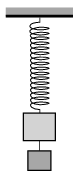Mathematical and Physical Journal
for High Schools
Issued by the MATFUND Foundation
 Already signed up? New to KöMaL?

#Problem P. 4991. (January 2018)

P. 4991. Two objects of total mass 4 kg, one of them is hanging below the other and attached to it by means of a thread, are attached to the lower end of a spring, hung onto a stand, as shown in the figure. If the lower object falls down the other one at the end of the spring begins to oscillate. If the two objects are interchanged and the lower one falls the other also begins to oscillate. The difference between the periods is 0.3 s. Calculate the mass of each object if the period of the oscillatory motion when both objects are on the spring is 1.5 s.(4 pont)

Deadline expired on February 12, 2018.

Sorry, the solution is available only in Hungarian. Google translation

Megoldás. Legyen az egyik test tömege $\displaystyle xM$, a másiké $\displaystyle (1-x)M$, a két test össztömege pedig $\displaystyle M=4$ kg. A rezgésidő a tömeg négyzetgyökével arányos, így fennáll:

$\displaystyle \sqrt{x}-\sqrt{1-x}=\frac{0{,}3}{1{,}5}.$

Négyzetre emelve mindkét oldalt:

$\displaystyle x+(1-x)-2\sqrt{x(1-x)}=\frac{1}{25},$

vagyis

$\displaystyle \sqrt{x(1-x)}= \frac{12}{25}.$

Ismét négyzetre emelve, majd a másodfokú egyenletet megoldva $\displaystyle x_1=\frac{16}{25}$ és $\displaystyle x_2=\frac{9}{25}$, vagyis a keresett tömegekre $\displaystyle m_1=2{,}56$ kg és $\displaystyle m_1=1{,}44$ kg adódik.

### Statistics:

 81 students sent a solution. 4 points: 70 students. 3 points: 9 students. 2 points: 1 student. 0 point: 1 student.

Problems in Physics of KöMaL, January 2018